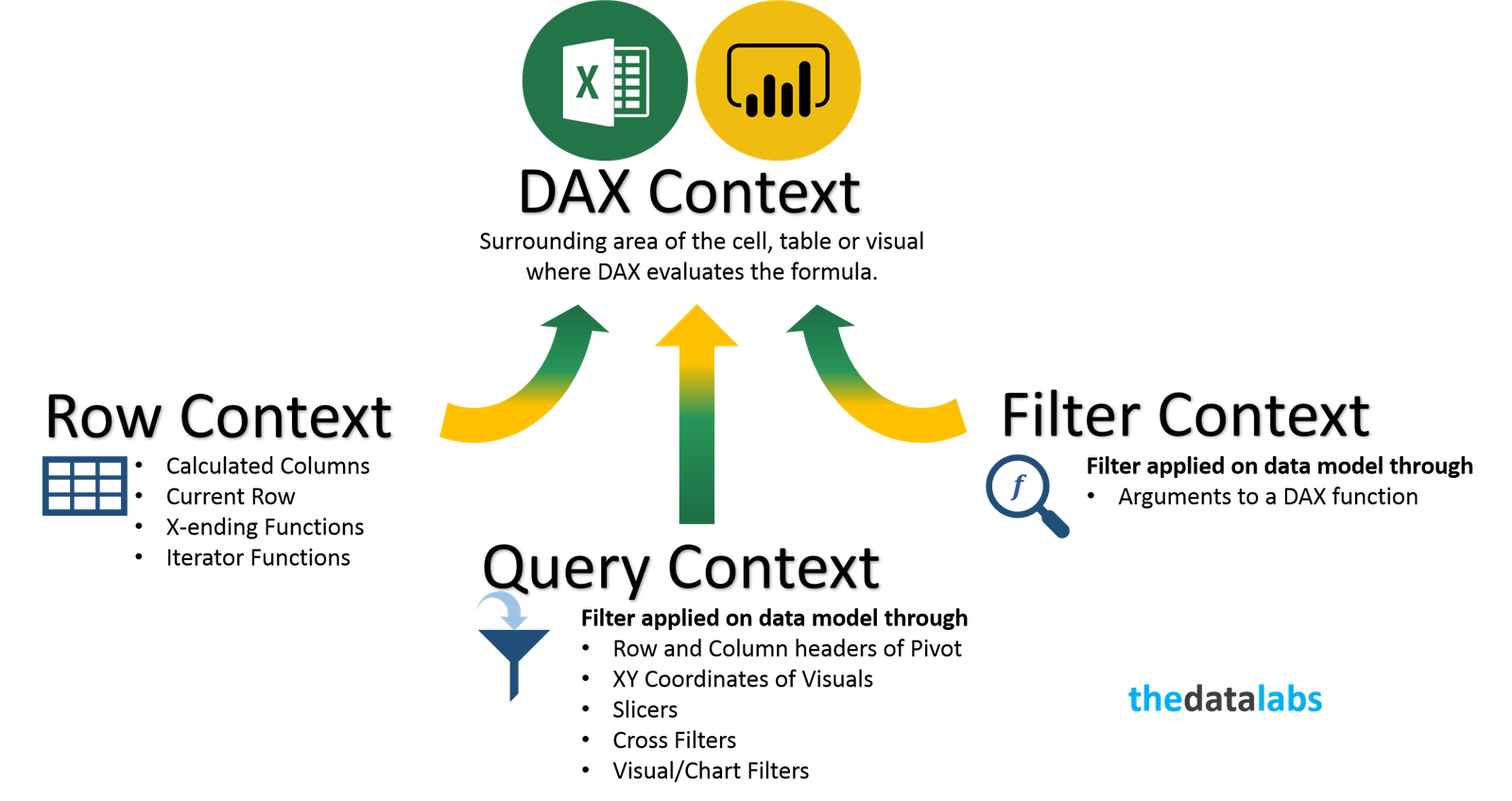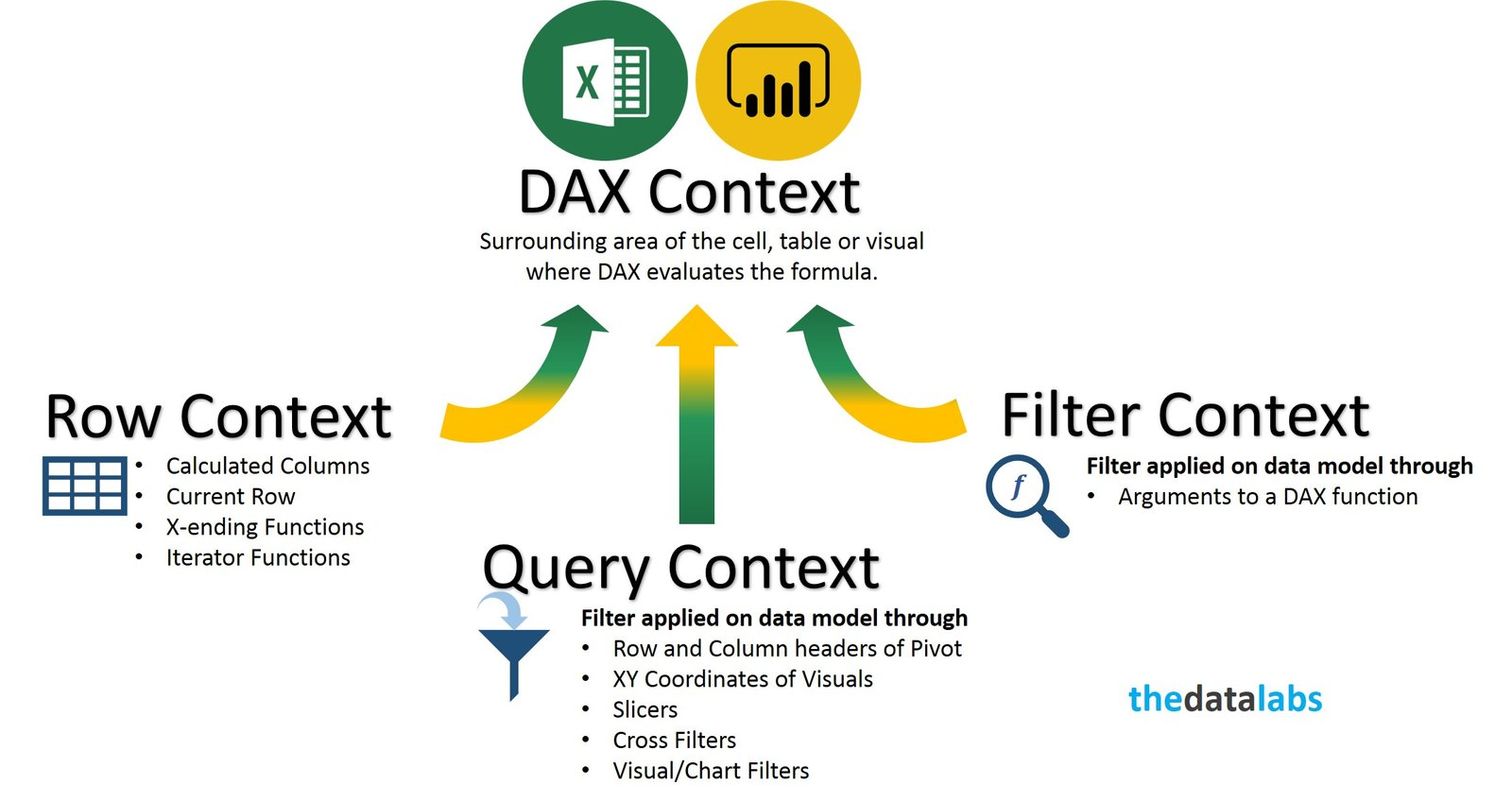# Filter Context### Understanding Evaluation Context in DAXIn simple language, we can say that the context is the “environment” under which the formula is evaluated. You can think of the evaluation context of a formula as the surrounding area of the cell where DAX evaluates the formula.

Context plays an important role in DAX and it enables you to perform dynamic analysis, in which the results of a formula can change to reflect the current row or cell selection and also any related data. Understanding context and using context effectively are very important for building high-performing formulas, dynamic analysis, and for troubleshooting problems in formulas.

Understanding context and using context effectively are very important for building high-performing formulas, dynamic analyses, and for troubleshooting problems in formulas.

### Types of Context in DAX

There are three different types of context: row context, query context, and filter context.### Row Context

A Row Context is a context that always contains a single row and DAX automatically defines it during the creation of calculated columns. Row context can be thought of as “the current row.”

If you have created a calculated column, the row context consists of the values in each individual row and values in columns that are related to the current row.

For example, suppose you create a calculated column, =[Freight] + [Tax],

, that adds together two columns from the same table. This formula behaves like formulas in an Excel table, which automatically reference values from the same row.

Row context does not interact with relationship automatically. To follow the relationships between tables to determine which rows in related tables are associated with the current row, we need to use RELATED and RELATEDTABLE functions in DAX. We will learn about these functions in our future posts.

For example, the following formula uses the RELATED function to fetch a tax value from a related table, based on the region that the order was shipped to. The tax value is determined by using the value for region in the current table, looking up the region in the related table, and then getting the tax rate for that region from the related table.

= [Freight] + RELATED(‘Region'[TaxRate])

This formula simply gets the tax rate for the current region, from the Region table. You do not need to know or specify the key that connects the tables.

Note: Row Context can also be created with some DAX functions (X-ending function and others) which we will discuss in upcoming posts.

### Query Context

Query context refers to the subset of data that is implicitly retrieved for a formula. When you drop a DAX measure or other value field into a cell in a PivotTable, Table and Visuals; the DAX engine examines the Row and Column headers, XY coordinates, Slicers, and Report filters to determine the context. Then, DAX engine makes the necessary calculations to populate value for each cell in the PivotTable, Tables and XY coordinates of Charts/Visuals. The set of data that is retrieved is the ‘Query Context’ for each cell.

Because the context can change – depending on where you place the formula, the results of the formula also change depending on whether you use the formula in a PivotTable, Table or Visuals with many groupings, filters (slicers and/or cross filter option), or in a calculated column with no filters and minimal context.

For example, suppose you create this simple formula that sums the values in the Profit column of the Sales table:=SUM(‘Sales'[Profit]).

If you use this formula in a calculated column within the Sales table, the results for the formula will be the same for the entire table, because the query context for the formula is always the entire data set of the Sales table. Your results will have profit for all regions, all products, all years, and so on.

In Power BI and Excel, it is easy to change context by adding or removing column and row headers, by adding or removing slicers and activating/deactivating visual interactions. You can create a formula like the one above, in a measure, and then drop it into a PivotTable or any Visuals/Charts. Whenever you add column or row headings to the PivotTable and additional categories on XY coordinates of any Visual/charts, you change the query context in which the measure is evaluated. Slicing and filtering operations also affect context. Therefore, the same formula, used in a PivotTable or Visuals, is evaluated in a different query context for each cell.

### Filter Context

Filter context is added when you specify filter constraints on the set of values allowed in a column or table, by using arguments to a DAX function. Filter context applies on top of other contexts, such as row context or query context.

For example, a PivotTable calculates its values for each cell based on the row and column headings, as described in the preceding section on query context. However, within the measures or calculated columns that you add to the PivotTable, you can specify filter expressions to control the values that are used by the formula. You can also selectively clear the filters on particular columns.

There are several filter functions available in DAX. Most of them are very complex and powerful. The filtering functions let you manipulate data context to create dynamic calculations. Some of filter functions are ALL, ALLEXCEPT, ALLSELECTED, CALCULATE, CALCULATETABLE and FILTERS etc.

### Filter Context and Relationships

By default, Row context does not interact with relationships automatically. If you want to traverse relationships, you have two different functions (RELATED and RELATEDTABLE) to use, depending on which side of the relationship you are on while accessing the target table. If you want to get the value from many-side on the one side then you can use RELATEDTABLE and if you want to get the value from one side on the many side then you can utilize RELATED function.

Query and Filter contexts behave in a different way. They don’t need any DAX functions to interact with relationships and it happens automatically. They have different behaviors depending on how you set the filtering of the relationship. The general rule is that the Query and Filter context propagates through a relationship if the filtering direction set on the relationship itself makes propagation feasible.